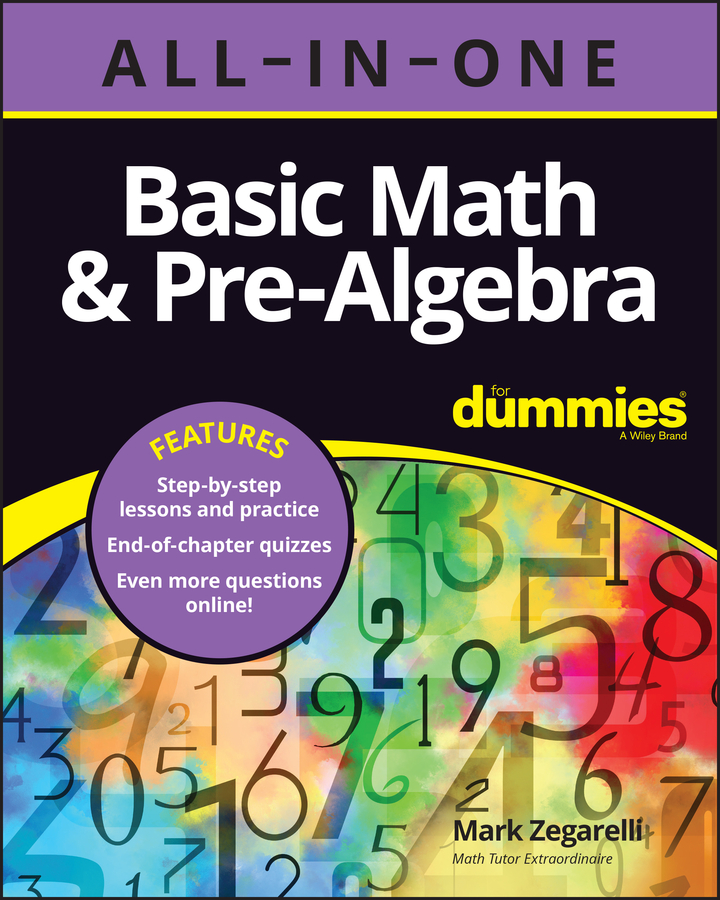##### Basic Math & Pre-Algebra All-in-One For Dummies (+ Chapter Quizzes Online)To work with sets, you need to understand terms such as elements and cardinality. You also need to know how to recognize equal sets, subsets, and empty sets, and how they relate to each other.

The things contained in a set are called elements (also known as members). Consider the following two sets:

A = {Empire State Building, Eiffel Tower, Roman Colosseum}

B = {Albert Einstein’s intelligence, Marilyn Monroe’s talent, Joe DiMaggio’s athletic ability, Senator Joseph McCarthy’s ruthlessness}

The Eiffel Tower is an element of A, and Marilyn Monroe’s talent is an element of B. You can write these statements using the symbol , which means is an element of:

Eiffel Tower A

Marilyn Monroe’s talent B

However, the Eiffel Tower is not an element of B. You can write this statement using the symbol , which means is not an element of:

Eiffel Tower B

These two symbols become more common as you move higher in your study of math.

Now let’s look at what’s inside those braces and how some sets relate to each other.

## Cardinality of sets

The cardinality of a set is just a fancy word for the number of elements in that set.

When A is {Empire State Building, Eiffel Tower, Roman Colosseum}, it has three elements, so the cardinality of A is three. Set B, which is {Albert Einstein’s intelligence, Marilyn Monroe’s talent, Joe DiMaggio’s athletic ability, Senator Joseph McCarthy’s ruthlessness}, has four elements, so the cardinality of B is four.

## Equal sets

If two sets list or describe the exact same elements, the sets are equal (you can also say they’re identical or equivalent). The order of elements in the sets doesn’t matter. Similarly, an element may appear twice in one set, but only the distinct elements need to match.

Suppose you define some sets as follows:

C = the four seasons of the year

D = {spring, summer, fall, winter}

E = {fall, spring, summer, winter}

F = {summer, summer, summer, spring, fall, winter, winter, summer}

Set C gives a clear rule describing a set. Set D explicitly lists the four elements in C. Set E lists the four seasons in a different order. And set F lists the four seasons with some repetition. Thus, all four sets are equal. As with numbers, you can use the equal sign to show that sets are equal:

C = D = E = F

## Subsets

When all the elements of one set are completely contained in a second set, the first set is a subset of the second. For example, consider these sets:

C = {spring, summer, fall, winter}

G = {spring, summer, fall}

As you can see, every element of G is also an element of C, so G is a subset of C. The symbol for subset is , so you can write the following:

G C

Every set is a subset of itself. This idea may seem odd until you realize that all the elements of any set are obviously contained in that set.

## Empty sets

The empty set — also called the null set — is a set that has no elements:

H = {}

As you can see, H is defined by listing its elements, but no elements are listed, so H is empty. The symbol is used to represent the empty set. So H = .

You can also define an empty set using a rule. For example,

I = types of roosters that lay eggs

Clearly, roosters are male and therefore can’t lay eggs, so this set is empty.

You can think of as nothing. And because nothing is always nothing, there’s only one empty set. All empty sets are equal to each other, so in this case, H = I.

Furthermore, is a subset of every other set, so the following statements are true:

A

B

C

This concept makes sense when you think about it. Remember that has no elements, so technically speaking, every element in is in every other set.